## Effect of temperature on PN diode characteristics:

Temperature on PN diode characteristics is very important consideration in the analysis or design of electronic devices or systems. It affects virtually all of the characteristics of a semiconductor device.

The diode current at a given voltage depends upon the operating temperature. The variation may be determined from Eq. (7.20), where the temperature is contained implicitly in VT and also in the reverse saturation current I0.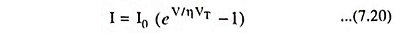From Eq. (7.20)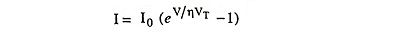At room temperature VT = 26 mV = 0.026 V

For germanium η = 1 and for silicon η = 2

So substituting the above values in the expression for diode current we have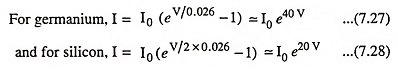The dependence of I0 on temperature T is, from Eqs. (7.19) and (7.21), given approximately as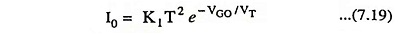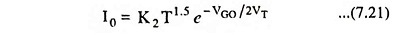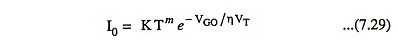where K is a constant and eVGO is the forbidden energy gap in joules

For silicon : η = 2; m = 1.5; VGO = 1.21 V

For germanium : η = 1; m = 2; VGO = 0.785 V

Taking the logarithm on both sides of Eq. (7.29), we have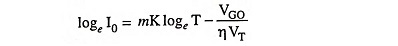Taking the derivative of the above equation we have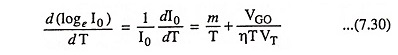At room temperature (300 K), from Eq. (7.30)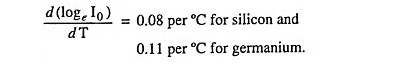The performance of commercial diodes is only consistent with above result. The reason for the discrepancy is that, in a practical diode, there is a component of the reverse saturation current due to leakage over the surface that has not been taken into account in Eq. (7.29). Since leakage component is independent of temperature, it may be expected to find a smaller rate of change of I0 with temperature than that predicted above.

From experimental data it is found that reverse saturation current I0 increases 7 per cent per °C for both silicon and germanium. Since (1.07)10 ≈2.0, the reverse saturation current approximately doubles for every 10°C rise in temperature.

Germanium is more temperature dependent than silicon because its reverse saturation current is approximately 1,000 times larger.

The change in V-I characteristics of a semiconductor diode due to variations in temperature above and below room temperature on PN diode characteristics is shown in Fig. 7.18.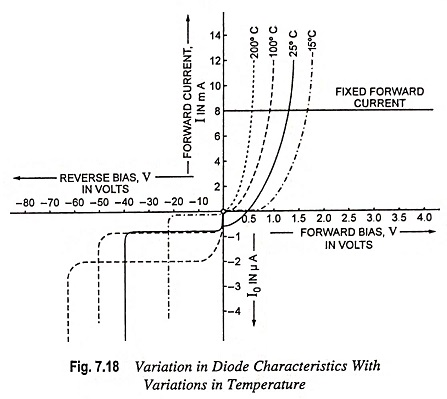From Fig. 7.18 we see that for a fixed level of forward voltage, forward current increases with the increase in temperature. If the forward current is measured, at the increased temperature, for several levels of forward voltage and the results are plotted, it is seen that current-voltage characteristic is moved to the left. The horizontal line on Fig. 7.18 shows that for a fixed forward current, forward voltage drops as the junction temperature increases i.e., forward voltage has a negative temperature coefficient. It is found that the temperature coefficient for the forward voltage of a P-N junction is approximately -1.8 mV/°C for Si and -2.02 mV/ °C for Ge. Zener potential is also experiencing a pronounced change in level.

Scroll to Top